# ABC Enterprises has five jobs that must be scheduled for production at a work center. Below...

ABC Enterprises has five jobs that must be scheduled for production at a work center. Below are the processing times and due dates for each job.

 Job Processing Time (in days) Due Date (days from today) 1 7 14 2 5 21 3 3 10 4 2 7 5 6 9

Schedule the jobs by sequencing them using the Earliest Due Date (EDD) priority rule.
What is the average flow time? Show your Work and round your answer to one decimal point.

 Job Processing Time (in days) Due Date (days from today) Flow Time

Schedule the jobs by sequencing them using the Shortest Processing Time (SPT) priority rule.
What is the average flow time? Show your Work and round your answer to one decimal point.

 Job Processing Time (in days) Due Date (days from today) Flow Time

EDD

 JOB PROCESSING TIME DUE TIME FLOW TIME JOB LATENESS D 2 7 0 + 2 = 2 E 6 9 2 + 6 = 8 C 3 10 8 + 3 = 11 1 A 7 14 11 + 7 = 18 4 B 5 21 18 + 5 = 23 2

TOTAL FLOW TIME = 2 + 8 + 11 + 18 + 23 = 62
AVERAGE COMPLETION TIME = FLOW TIME / N = 62 / 5 = 12.4

SPT

 JOB PROCESSING TIME DUE TIME FLOW TIME JOB LATENESS D 2 7 0 + 2 = 2 C 3 10 2 + 3 = 5 B 5 21 5 + 5 = 10 E 6 9 10 + 6 = 16 7 A 7 14 16 + 7 = 23 9

TOTAL FLOW TIME = 2 + 5 + 10 + 16 + 23 = 56
AVERAGE COMPLETION TIME = FLOW TIME / N = 56 / 5 = 11.2

** Leaving a thumbs-up would really help me out. Let me know if you face any problems.

#### Earn Coin

Coins can be redeemed for fabulous gifts.

Similar Homework Help Questions
• ### a) Using the EDD (earliest due date) decision rule for sequencing the jobs, the order is...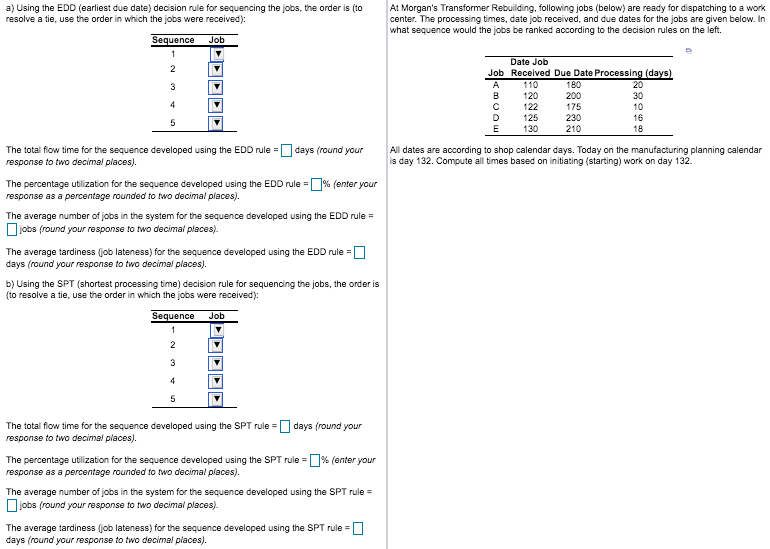a) Using the EDD (earliest due date) decision rule for sequencing the jobs, the order is (to resolve a tie, use the order in which the jobs were received): At Morgan's Transformer Rebuilding, following jobs (below) are ready for dispatching to a work center. The processing times, date job received, and due dates for the jobs are given below. In what sequence would the jobs be ranked according to the decision rules on the left Sequence Job 1 Date Job...

• ### a) Using the EDD (earliest due date) decision rule for sequencing the jobs, the order is...a) Using the EDD (earliest due date) decision rule for sequencing the jobs, the order is (to resolve a tie, use the order in which the jobs were received): At Morgan's Transformer Rebuilding, following jobs (below) are ready for dispatching to a work center. The processing times, date job received, and due dates for the jobs are given below. In what sequence would the jobs be ranked according to the decision rules on the left Sequence Job 1 Date Job...

• ### 4. [10 points] Today is Nov 15. The following jobs need to be scheduled on the...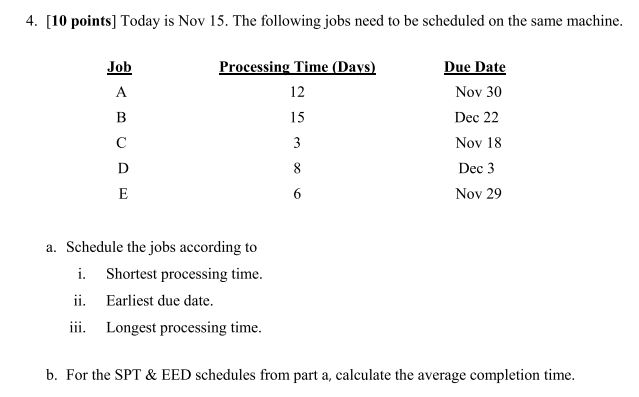4. [10 points] Today is Nov 15. The following jobs need to be scheduled on the same machine. Job Processing Time (Davs) Due Date Nov 30 Dec 22 Nov 18 Dec 3 Nov 29 12 a. Schedule the jobs according to i. Shortest processing time ii. Earliest due date. iii. Longest processing time. b. For the SPT& EED schedules from part a, calculate the average completion time.

• ### ​a) Using the SPT​ (shortest processing​ time) decision rule for sequencing the​ jobs, the order is​...

​a) Using the SPT​ (shortest processing​ time) decision rule for sequencing the​ jobs, the order is​ (to resolve a​ tie, use the order in which the jobs were​ received): Sequence Job 1 ▼ N L M K O 2 ▼ K O L M N 3 ▼ K N L O M 4 ▼ N L M O K 5 ▼ N O K M L The total flow time for the sequence developed using the SPT rule​ = nothing...

• ### Problem 1: A forensic audit firm has five audit jobs remaining at a point in time....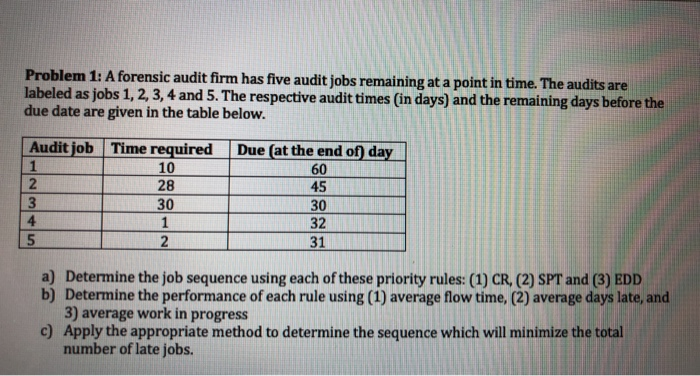Problem 1: A forensic audit firm has five audit jobs remaining at a point in time. The audits are labeled as jobs 1, 2, 3, 4 and 5. The respective audit times (in days) and the remaining days before the due date are given in the table below. | Audit job Time required 10 28 30 Due (at the end of day 60 45 30 32 31 le a) Determine the job sequence using each of these priority rules: (1)...

• ### 5. A work center has five jobs assigned to it. They are labeled, in the order...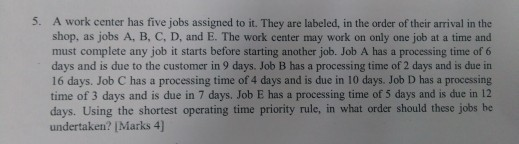5. A work center has five jobs assigned to it. They are labeled, in the order of their arrival in the shop, as jobs A, B, C, D, and E. The work center may work on only one job at a time and must complete any job it starts before starting another job. Job A has a processing time of 6 days and is due to the customer in 9 days. Job B has a processing time of 2 days...

• ### Question 20, Math problem 20. The Mowry Machine Shop still has five jobs to be pro-...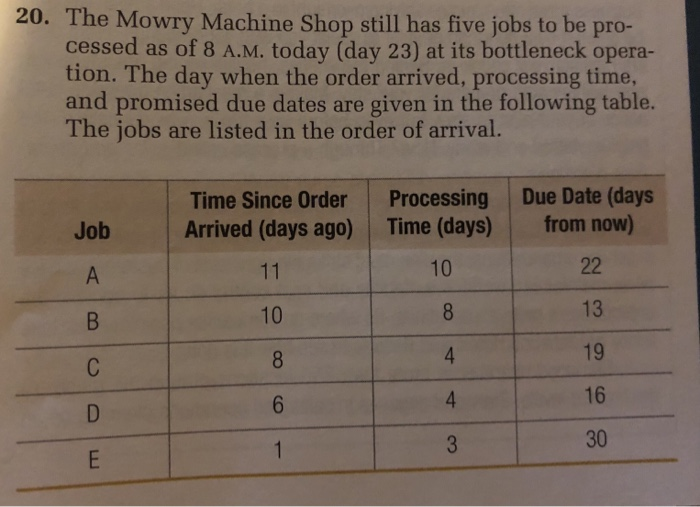Question 20, Math problem 20. The Mowry Machine Shop still has five jobs to be pro- cessed as of 8 A.M. today (day 23) at its bottleneck opera- tion. The day when the order arrived, processing time, and promised due dates are given in the following table. The jobs are listed in the order of arrival. Time Since Order Arrived (days ago) Processing Time (days) Due Date (days from now) Job А 11 10 22 10 8 В 13 8...

• ### Problem 2 (6 points): Refer to the following data for jobs waiting to be processed at...

Problem 2 (6 points): Refer to the following data for jobs waiting to be processed at a single work center (jobs are shown in order of arrival): Job Processing Time (Days) Due Date (days from Now) A 2 2 B 5 6 C 6 10 D 9 6 E 4 8 What is the average flow time for the shortest processing time (SPT) rule schedule?

• ### Using the FCFS (first come, first served) decision rule for sequencing the jobs, the order is:...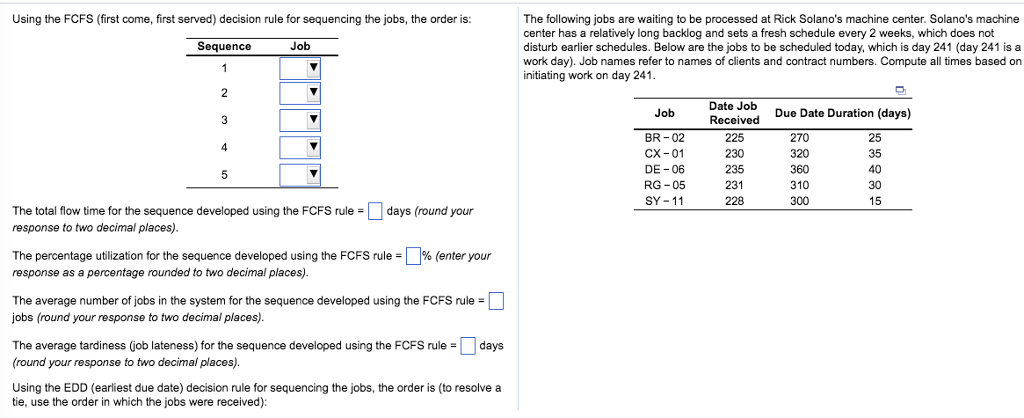Using the FCFS (first come, first served) decision rule for sequencing the jobs, the order is: The following jobs are waiting to be processed at Rick Solano's machine center. Solano's machine center has a relatively long backlog and sets a fresh schedule every 2 weeks, which does not disturb earlier schedules. Below are the jobs to be scheduled today, which is day 241 (day 241 is a work day). Job names refer to names of clients and contract numbers. Compute...

• ### decimal places). An Alabama lumberyard has four jobs on order, as shown in the following table....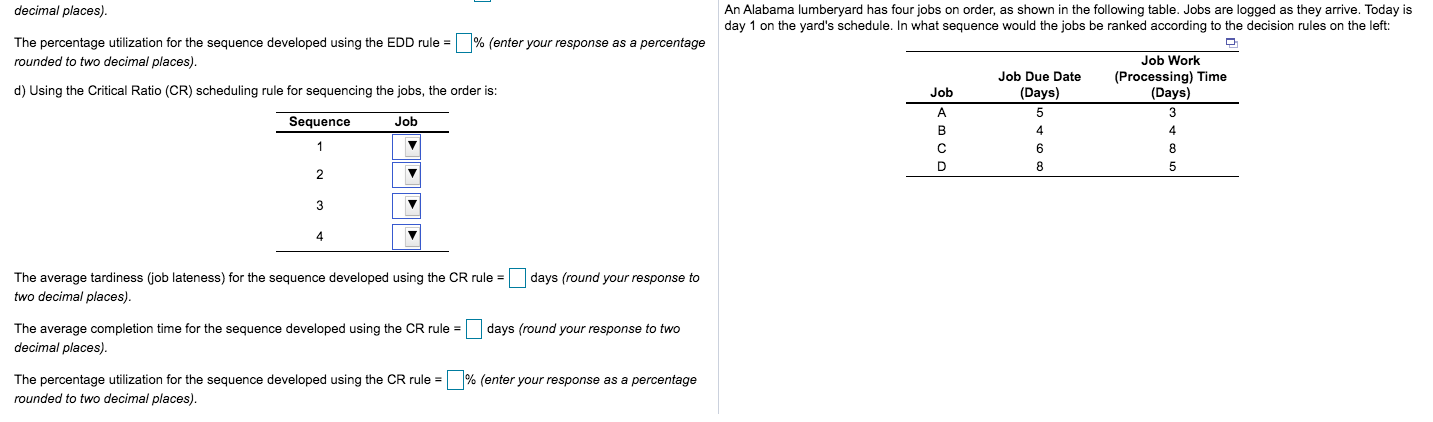decimal places). An Alabama lumberyard has four jobs on order, as shown in the following table. Jobs are logged as they arrive. Today is day 1 on the yard's schedule. In what sequence would the jobs be ranked according to the decision rules on the left: % (enter your response as a percentage The percentage utilization for the sequence developed using the EDD rule = rounded to two decimal places). Job Work (Processing) Time (Days) Job Due Date (Days) d)...